# Calculating Future Date

This post will guide you how to calculate a future date based on a given data in excel. How do I add one or more days to a given date to calculate a future date with a formula in excel. How to calculate a future date excluding weekends in excel. How to calculate a future date excluding weekends and holidays based on a given date with formula in excel.

## Calculating Future Date

Assuming that you have a date list in the range of cells B1:B4, and you want to add any number of days (50) into those dates to get a new future new date. You can type this formula into a blank cell in column C.

`=B2+50`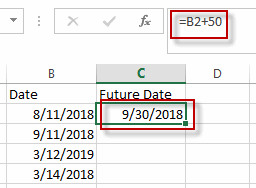Then you need to press enter key in your keyboard, and drag the AutoFill Handler over other cells to apply this formula to add number 50 to get the future dates.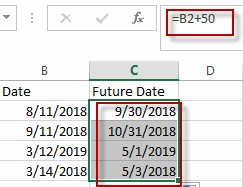## Calculating Future Date Excluding Weekends

If you want to calculate a new future date excluding weekends, and you need to use the WORKDAY function to create a new formula to exclude all weekends in the future date. So you can write down this formula:

`=WORKDAY(B2,50)`

#1 Type this formula into the formula box of the cell C2, and then press Enter key in your keyboard.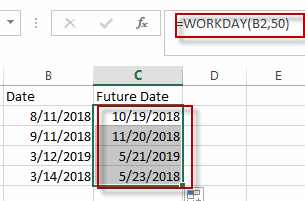#2 go to HOME tab, click Short Date from the General drop down menu list.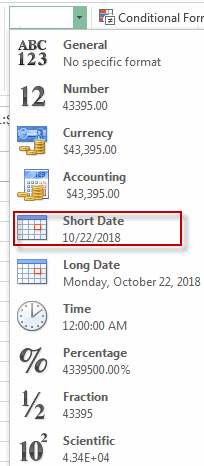## Calculating Future Date Excluding Weekends and Holidays

If you want to calculate a future date excluding weekends and holidays based on a given date, you still need to use the WORKDAY function to create a new formula. At this time, you need to specify which dates are holidays in the certain range of cells (such as: E1:E3).

You can use the following formula to achieve the result:

`=WORKDAY(B2,50,\$E\$1:\$E\$3)`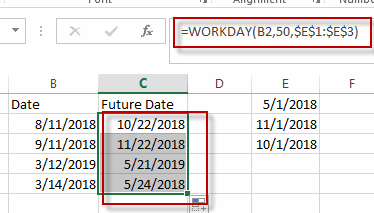Then you can go to HOME tab, click Short Date from the General drop down menu list.so the date can be converted as a short date from a five-digit number.

### Related Functions

• Excel WORKDAY Function
The Excel WORKDAY function returns a serial number that represents a date that is the indicated number of working days before or after the starting date you specified. The syntax of the WORKDAY function is as below:=WORKDAY(start_date, days, [holidays])…